Gauge your potential with Aakash BYJU'S JEE Main'23 Mock Test Gauge your potential with Aakash BYJU'S JEE Main'23 Mock Test

# JEE Main 2021 Physics Paper With Solutions Feb 25 Shift 1

JEE Main 2021 Feb 25 Shift 1 Physics question paper with solutions is available here for instant download. The question paper comes with solutions that have been extensively prepared by our subject-matter experts. The detailed solutions will facilitate easy learning and students will gain a clear understanding of the concepts covered in the question paper. In addition, students will be able to grasp the answers easily and they will also be able to get a much clearer idea of how the Physics paper was set, the types of questions framed, marking scheme, duration of the paper and more.
Students can download and use the JEE Main 2021 Feb 25 Shift 1 Physics question paper as a practice study material and start solving the questions without checking the answers first. With this, students will further be able to test their preparation level. While solving the questions students can revise the different topics before the main exam. All in all, working with the JEE Main 2021 Physics question paper will enable students to prepare productively and even achieve a high score in the actual exam.

### JEE Main 2021 25th February Shift 1 Physics Question Paper

SECTION A

Question 1: A 5V battery is connected across the points X and Y. Assume D1 and D2 to be normal silicon diodes. Find the current supplied by the battery if the +ve terminal of the battery is connected to point X.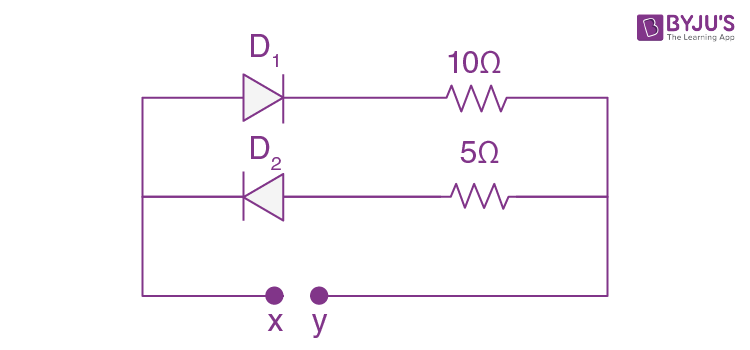1. a. ~0.86 A
2. b. ~0.5 A
3. c. ~0.43 A
4. d. ~1.5 A

Solution:

Since silicon diode is used so 0.7 Volt is drop across it, only D1 will conduct so current through cell.

I = (5 - 0.7)/10 = 0.43 A

Question 2: A solid sphere of radius R gravitationally attracts a particle placed at 3R from its centre with a force F1. Now a spherical cavity of radius R/2 is made in the sphere (as shown in figure) and the force becomes F2. The value of F1: F2 is: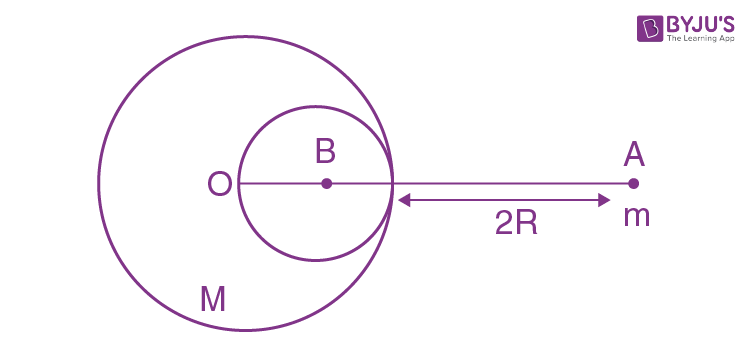1. a. 41 : 50
2. b. 36 : 25
3. c. 50 : 41
4. d. 25 : 36

Solution:

Gravitational field intensity g1 = GM/(3R)2 = GM/9R2 …(1)

Gravitational field intensity g2 = GM/9R2 - G(M/8)/(5R/2)2

= GM/9R2 - GM/R250

= (41/9×50) GM/R2….(2)

Implies , g1/g2 = 41/50

⇒ F1/F2 = mg1/mg2 = 41/50

Question 3: A student is performing the experiment of resonance column. The diameter of the column tube is 6 cm. The frequency of the tuning fork is 504 Hz. Speed of the sound at the given temperature is 336 m/s. The zero of the metre scale coincides with the top end of the resonance column tube. The reading of the water level in the column when the first resonance occurs is:

1. a. 13 cm
2. b. 14.8 cm
3. c. 16.6 cm
4. d. 18.4 cm

Solution:

λ = v/f

= 336/504 = 66.66cm

λ/4 = l + e = l + 0.3d

= l + 1.8

16.66 = l + 1.8 cm

l = 14.86 cm

Question 4: A diatomic gas, having Cp = (7/2)R and Cv = (5/2)R, is heated at constant pressure. The ratio dU : dQ : dW

1. a. 3 : 7 : 2
2. b. 5 : 7 : 2
3. c. 5 : 7 : 3
4. d. 3 : 5 : 2

Solution:

Since the gas is diatomic in nature and the process is isobaric, we have

Cp = (7/2)R

Cv = (5/2)R

dU = nCvdT

dQ = nCpdT

dW = nRdT

dU : dQ : dW

Cv : Cp : R

(5/2) R : (7/2) R : R

5 : 7 : 2

Question 5: Statement I: A speech signal of 2 kHz is used to modulate a carrier signal of 1 MHz. The bandwidth requirement for the signal is 4 kHz.

Statement II: The side band frequencies are 1002 kHz and 998 kHz.

In the light of the above statements, choose the correct answer from the options given below:

1. a. Both statement I and statement II are false
2. b. Statement I is false but statement II is true
3. c. Statement I is true but statement II is false
4. d. Both statement I and statement II are true

Solution:

Side band = (fc - fm) to (fc + fm)

= (1000 - 2) kHz to (1000 + 2) kHz

= 998 kHz to 1002 kHz

Band width = 2fm

= 2 ×2kHz

= 4 kHz

Both statements are true.

Question 6: The current (i) at time t = 0 and t = ∞ respectively for the given circuit is: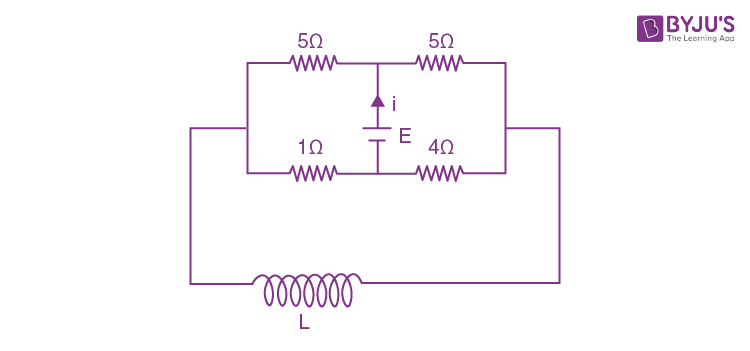1. a. 18E/55,5E/18
2. b. 5E/18,18E/55
3. c. 5E/18,10E/33
4. d. 10E/33,5E/18

Solution: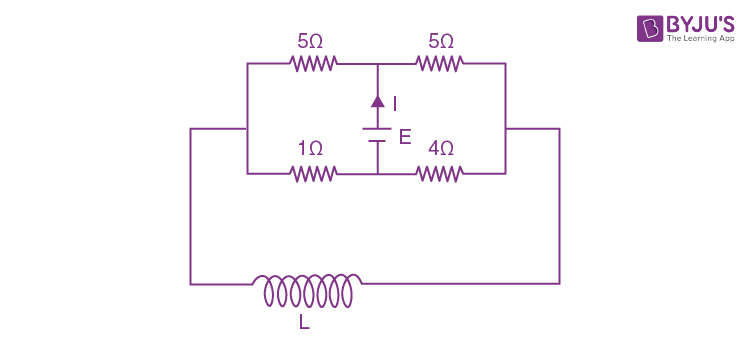At t = 0, inductor is open

Initial-state equivalent of the circuit shown in figure -1 is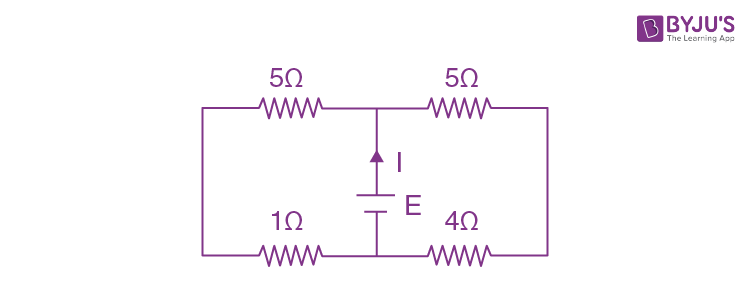Req = 6×9/(6+9) = 54/15

I (t = 0) = E×15/54 = 5E/18

At t = ∞, For steady state inductor is replaced by plane wire

Steady state equivalent of the circuit shown in figure-1 is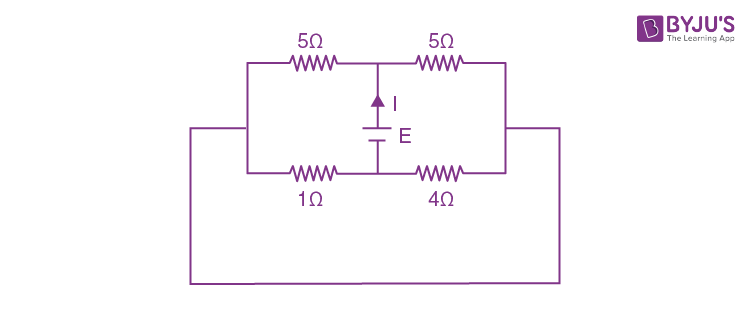Equivalent circuit diagram is given by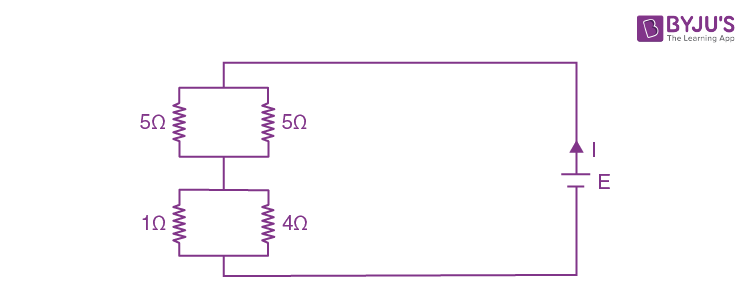Req =1×4/(1+4) + 5×5/(5+5)

= 4/5 + 5/2

= (8 + 25)/10

= 33/10

I = E/Req = 10E/33

Question 7: Two satellites A and B of masses 200 kg and 400 kg are revolving round the earth at height of 600 km and 1600 km respectively.

If TA and TB are the time periods of A and B respectively then the value of TB - TA:[Given: Radius of earth = 6400 km, mass of earth = 6×1024 kg]

1. a. 4.24× 102 s
2. b. 3.33 × 102 s
3. c. 1.33 × 103 s
4. d. 4.24 × 103 s

Solution:V = √(GMe/r)

$$T = \frac{2\pi r}{\sqrt{\frac{GM_{e}}{r}}} = 2\pi r\sqrt{\frac{r}{GM_{e}}}$$

T =√(4𝜋2r3/GMe) = √(4𝜋2r3/GMe)

T2 - T1 = √(4𝜋2(8000×103)3/G×6×1024 ) - √(4𝜋2(7000×103)3/G×6×1024 )

≅ 1.33 ×103 s

Question 8: An engine of a train, moving with uniform acceleration, passes the signal post with velocity u and the last compartment with velocity v. The velocity with which middle point of the train passes the signal post is:

1. a. √(v2 - u2)/2
2. b. (v - u)/2
3. c. √(v2 + u2)/2
4. d. (u + v)/2

Solution:a = uniform acceleration

u = velocity of first compartment

v = velocity of last compartment

l = length of train

v2 = u2 + 2as (3rd equation of motion)

v2 = u2 + 2al .....(1)

v2middle = u2 + 2al/2

∴ v2middle = u2 + al ....(2)

From equation (1) and (2)

v2middle = u2 + (v2 - u2)/2

= (v2 + u2)/2

∴ vmiddle = √(v2 + u2)/2

Question 9: A proton, a deuteron and an α particle are moving with the same momentum in a uniform magnetic field. The ratio of magnetic forces acting on them is _______ and their speeds are in the ratio______.

1. a. 2 : 1 : 1 and 4 : 2 : 1
2. b. 1 : 2 : 4 and 2 : 1 :1
3. c. 1 : 2 : 4 and 1 : 1 : 2
4. d. 4 : 2 : 1 and 2 : 1 : 1

Solution:

As v = p/m & F = qvB

∴ F = qpB/m

F1 = qpB/m, v1 = p/m

F2 = qpB/2m, v2 = p/2m

F3 = 2qpB/4m, v3 = p/4m

F1 : F2 : F3 & V1 : V2 : V3

1 : ½ : 1/2 & 1 : ½ :1/4

2 : 1 : 1 & 4 : 2 : 1

Question 10: Given below are two statements: one is labelled as Assertion A and the other is labelled as Reason R.

Assertion A: When a rod lying freely is heated, no thermal stress is developed in it.

Reason R: On heating, the length of the rod increases

In the light of the above statements, choose the correct answer from the options given below:

1. a. A is true but R is false
2. b. Both A and R are true and R is the correct explanation of A
3. c. Both A and R are true but R is NOT the correct explanation of A
4. d. A is false but R is true

Solution:

If a rod is free and it is heated then there is no thermal stress produced in it.

The rod will expand due to increase in temperature.

So, both A & R are true.

Question 11: In an octagon ABCDEFGH of equal side, what is the sum of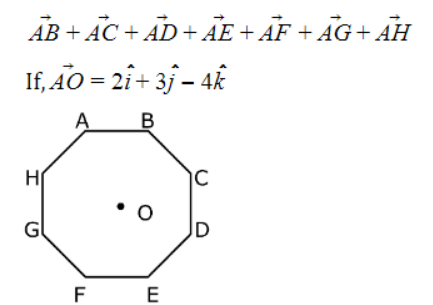1. a.
$$16\hat{i} + 24\hat{j} - 32\hat{j}$$
2. b.
$$-16\hat{i} - 24\hat{j} - 32\hat{j}$$
3. c.
$$-16\hat{i} - 24\hat{j} + 32\hat{j}$$
4. d.
$$-16\hat{i} + 24\hat{j} + 32\hat{j}$$

Solution: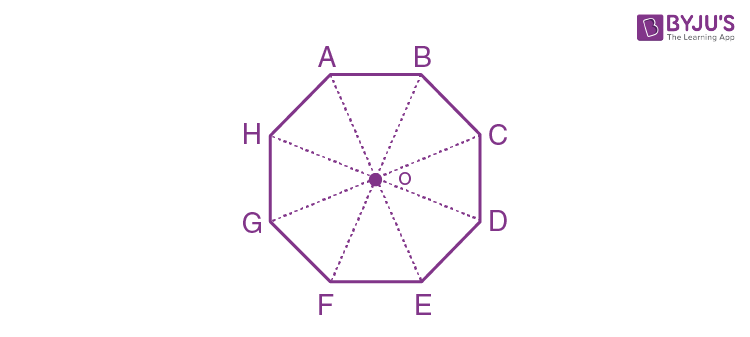Question 12: Two radioactive substances X and Y originally have N1 and N2 nuclei respectively. Half-life of X is half of the half-life of Y. After three half-lives of Y, numbers of nuclei of both are equal. The ratio N1/N2 will be equal to:

1. a. 8/1
2. b. 1/8
3. c. 3/1
4. d. 1/3

Solution:

After n half-life no of nuclei undecayed = No/2n

Given, t(1/2)x= t(1/2y)/2

So 3 half-life of y = 6 half-life of x

Given, Nx = Ny after 3t(½)y

N1/26 = N2/23

N1/N2 = 26/23 = 23= 8/1

Question 13: Match List-I with List-II:

 List-I List-II (a)h (Planck’s constant) (i) [MLT–1] (b)E (Kinetic energy) (ii) [ML2T–1] (c)V (Electric potential) (iii) [ML2T–2] (d)P (Linear momentum) (iv) [ML2I–1T–3]

Choose the correct answer from the options given below:

1. a. (a) → (ii), (b) →(iii), (c) → (iv), (d) → (i)
2. b. (a) →(i), (b) → (ii), (c) →(iv), (d) → (iii)
3. c. (a) → (iii), (b) → (ii), (c) →(iv), (d) →(i)
4. d. (a) → (iii), (b) → (iv), (c) →(ii), (d) →(i)

Solution:

K.E. = [ML2T–2]

P (Linear momentum) = [MLT–1]

h (Planck’s constant) = [ML2T–1]

V (Electric potential) = [ML2T–3I–1]

Question 14: The pitch of the screw gauge is 1 mm and there are 100 divisions on the circular scale. When nothing is put in between the jaws, the zero of the circular scale lines 8 divisions below the reference line. When a wire is placed between the jaws, the first linear scale division is clearly visible while 72nd division on circular scale coincides with the reference line. The radius of the wire is:

1. a. 1.64 mm
2. b. 1.80 mm
3. c. 0.82 mm
4. d. 0.90 mm

Solution:

Least count. = pitch/no. of div.

= 1 mm/100

= 0.01 mm

+ve zero error = 8 × L.C. = +0.08 mm

Measured reading = 1mm + 72 × L.C.

= 1mm + 0.72 mm

= 1.72 mm

True reading = 1.72 - 0.08

= 1.64 mm

Radius = 1.64/2 = 0.82 mm

Question 15: If the time period of a two meter long simple pendulum is 2 s, the acceleration due to gravity at the place where pendulum is executing S.H.M. is:

1. a. 2π2 ms–2
2. b. 16 m/s2
3. c. 9.8 ms–2
4. d. π2 ms–2

Solution:

T = 2π√(l/g)

T2 = 4π2l/g

g = 4π2l/T2

= 4π2×2/(2)2 = 2π2 ms–2

Question 16: An α particle and a proton are accelerated from rest by a potential difference of 200 V. After this, their de Broglie wavelengths are λα and λp respectively. The ratio λpα is:

1. a. 8
2. b. 2.8
3. c. 3.8
4. d. 7.8

Solution:

λ = h/p

= h/√(2mqV)

λpα = √(mαqα/mpqp)

= √(4×2/1×1)

= 2√2 = 2.8

Question 17: Given below are two statements: one is labelled as Assertion A and the other is labelled as reason R.

Assertion A: The escape velocities of planet A and B are same. But A and B are of unequal masses.

Reason R: The product of their masses and radii must be same. M1R1 = M2R2

In the light of the above statements, choose the most appropriate answer from the options given below:

1. a. Both A and R are correct but R is NOT the correct explanation of A
2. b. A is correct but R is not correct
3. c. Both A and R are correct and R is the correct explanation of A
4. d. A is not correct but R is correct

Solution:

Ve = escape velocity

ve = √(2GM/R)

So, for same ve, M1/R1 = M2/R2

A is true but R is false.

Question 18: The angular frequency of alternating current in a L-C-R circuit is 100 rad/s. The components connected are shown in the figure. Find the value of inductance of the coil and capacity of condenser.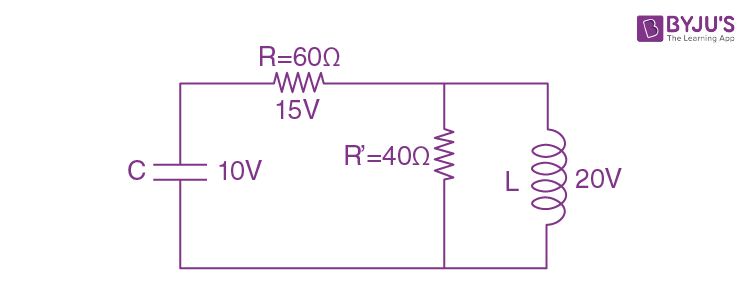1. a. 0.8 H and 250 μF
2. b. 0.8 H and 150 μF
3. c. 1.33 H and 250 μF
4. d. 1.33 H and 150 μF

Solution: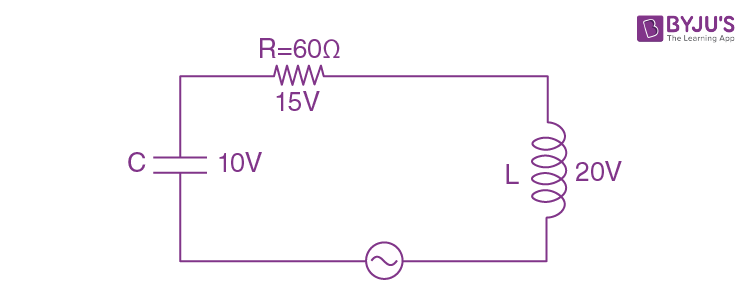Since key is open, circuit is series L-C-R circuit

15 = irms (60)

∴irms = ¼ A

Now, 20 = ¼ XL = ¼ (ωL)

∴ L = 4/5 = 0.8 H

& 10 = ¼ 1/(100C)

C = (1/4000) F

= 250 μF

Question 19: Two coherent light sources having intensities in the ratio 2x produce an interference pattern. The ratio (Imax - Imin)/(Imax + Imin) will be:

1. a. 2√(2x)/(x + 1)
2. b. √(2x)/(2x + 1)
3. c. 2√(2x)/(2x + 1)
4. d. √(2x)/(x + 1)

Solution:

Let I1 = 2x

I2 = 1

Imax = (√I1 + √I2)2

Imin = (√I1 - √I2)2

$$\frac{I_{max} - I_{min}}{I_{max} + I_{min}} = \frac{(\sqrt{2x}+ 1)^{2} - {(\sqrt2x - 1)^{2}}}{{(\sqrt2x + 1)^{2}} + {(\sqrt2x - 1)^{2}}}$$

= 4√(2x)/(2 + 4x)

= 2√(2x)/(2x + 1)

Question 20: Magnetic fields at two points on the axis of a circular coil at a distance of 0.05 m and 0.2 m from the centre are in the ratio 8 : 1. The radius of coil is ______

1. a. 0.15 m
2. b. 0.2 m
3. c. 0.1 m
4. d. 1.0 m

Solution: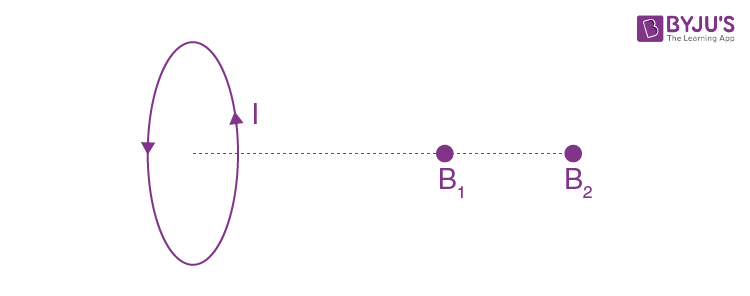B = μ0NiR2/2(R2 + x2)3/2

at x1 = 0.05m, B1 = μ0NiR2/2(R2 + (0.05)2)3/2

at x2 = 0.2m, B2 = μ0NiR2/2(R2 + (0.2)2)3/2

B1/B2 = (R2 + 0.04)3/2/(R2 + 0.0025)3/2

(8/1)2/3 = (R2 + 0.04)/(R2 + 0.0025)

4 (R2 + 0.0025) = R2 + 0.04

3R2 = 0.04 - 0.01

R2 = 0.03/3 = 0.01

R = √0.01 = 0.1 m

Section - B

Question 1: The same size images are formed by a convex lens when the object is placed at 20 cm or at 10 cm from the lens. The focal length of a convex lens is ______ cm.

Solution:

1/v – 1/u = 1/f ...(1)

m = v/u ....(2)

from (1) and (2) we get

m = f/(f + u)

Given conditions

m1 = -m2

f/(f - 10) = -f/(f - 20)

f – 20 = –f + 10

2f = 30

f = 15 cm

Question 2: The electric field in a region is given by

$$\left ( \frac{3}{5}E_{o}\hat{i}+ \frac{4}{5}E_{o}\hat{j}\right )\frac{N}{C}$$
The ratio of flux of reported field through the rectangular surface of area 0.2 m2 (parallel to y-z plane) to that of the surface of area 0.3 m2(parallel to x-z plane) is a : 2, where a = ________

[Here i, j and k are unit vectors along x, y and z-axes respectively]

Solution: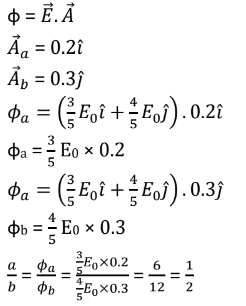Therefore, a = 1

Question 3: 512 identical drops of mercury are charged to a potential of 2 V each. The drops are joined to form a single drop. The potential of this drop is ____ V.

Solution:

Let charge on each drop = q

V = kq/r

2 = kq/r

4πR3/3 = 512 × (4/3) πr3

R = 8r

V = k(512)q/R = (512/8) (kq/r)

= (512/8) × 2

= 128 V

Question 4: The potential energy (U) of a diatomic molecule is a function dependent on r (interatomic distance) as U= α/r10- β/r5 - 3. Where, a and b are positive constants. The equilibrium distance between two atoms will (2α/β)a/b. Where a =_______

Solution:

F = -dU/dr

F = -[-10α/r11 + 5β/r6]

for equilibrium, F = 0

10α/r11 = 5β/r6

2α/β = r5

r = (2α/β)1/5

a = 1

Question 5: A small bob tied at one end of a thin string of length 1m is describing a vertical circle so that the maximum and minimum tension in the string are in the ratio 5 : 1. The velocity of the bob at the highest position is ______ m/s. (take g = 10 m/s2)

Solution: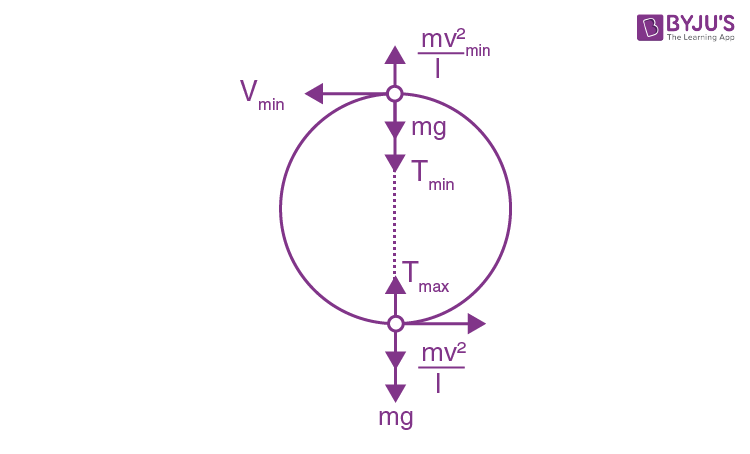By conservation of energy,

v2min = V2 – 4gl ....(1)

Tmax = mg + mv2/l ....(2)

Tmin = mv2min/l - mg ....(3)

from equation (1) and (3)

Tmin = (m/l) (v2 – 4gl) – mg

Tmax/Tmin = (v2/l + g)/(v2/l - 5g)

5/1 = (v2/1 + 10)/ (v2/1 - 50)

5v2 – 250 = v2 + 10

v2 = 65 ....(4)

from equation (4) and (1)

v2min = 65 - 40 = 25

vmin = 5 m/s

Question 6: In a certain thermodynamic process, the pressure of a gas depends on its volume as kV3. The work done when the temperature changes from 1000 C to 3000 C will be ____ nR, where n denotes number of moles of a gas.

Solution:

P = kv3

pv–3 = k

x = –3

w = nR(T1 - T2)/(x - 1)

= nR(100-300)/(-3 -1)

= nR(-200)/-4

= 50nR

Question 7: In the given circuit of potentiometer, the potential difference E across AB (10 m length) is larger than E1 and E2 as well. For key K1 (closed), the jockey is adjusted to touch the wire at point J1 so that there is no deflection in the galvanometer. Now the first battery (E1) is replaced by the second battery (E2) for working by making K1 open and E2 closed. The galvanometer gives then null deflection at J2. The value of E1/E2 is the smallest fraction of a/b, Then the value of a is ____.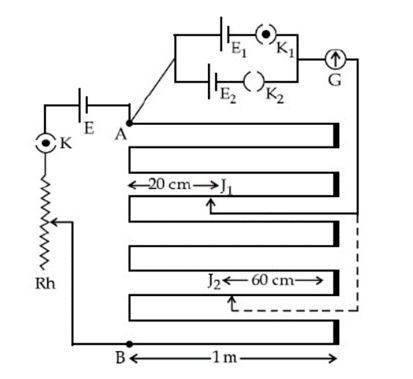Solution:

E1/E2 = l1/l2

= 3×100 cm + (100 - 20)cm)/(7×100 cm + 60 cm)

= 380/760

= ½

= a/b

a = 1

Question 8: A monoatomic gas of mass 4.0 u is kept in an insulated container. Container is moving with a velocity 30 m/s. If container is suddenly stopped then change in temperature of the gas (R=gas constant) is x/3R. Value of x is ______.

Solution:

ΔKE = ΔU

ΔU = nCVΔT

½ mv2 = (3/2) nRΔT

mv2/3nR = ΔT

4×(30)2/3×1×R = ΔT

ΔT = 4×(30)2/3×1×R

x/3R = 1200/R

x = 3600

Question 9: A coil of inductance 2 H having negligible resistance is connected to a source of supply whose voltage is given by V = 3t volt. (where t is in second). If the voltage is applied when t = 0, then the energy stored in the coil after 4 s is _______ J.

Solution:

L di/dt = ε

= 3t

L ∫di = 3 ∫t dt

Li = 3t2/2

i = 3t2/2L

Energy stored in the coil, E = ½ Li2

= ½ L (3t2/2L)2

= ½ × 9t4/4L

= 9/8 × (4)4/2

= 144 J

Question 10: A transmitting station releases waves of wavelength 960 m. A capacitor of 256 μF is used in the resonant circuit. The self inductance of coil necessary for resonance is _____ × 10–8H.

Solution:

At resonance

ωr = 1/√(Lc)

∴ 2πf = 1/√(Lc)

∴ 4π2c22 = 1/Lc

∴4π2×3×108×3×108/960×960 = 1/L×2.56×10-6

L = 375×960/10-6×4×π2×9×1016 = 103/1010

= 10–7 H

= 10 × 10–8 H

### JEE Main 2021 Physics Paper February 25 Shift 1